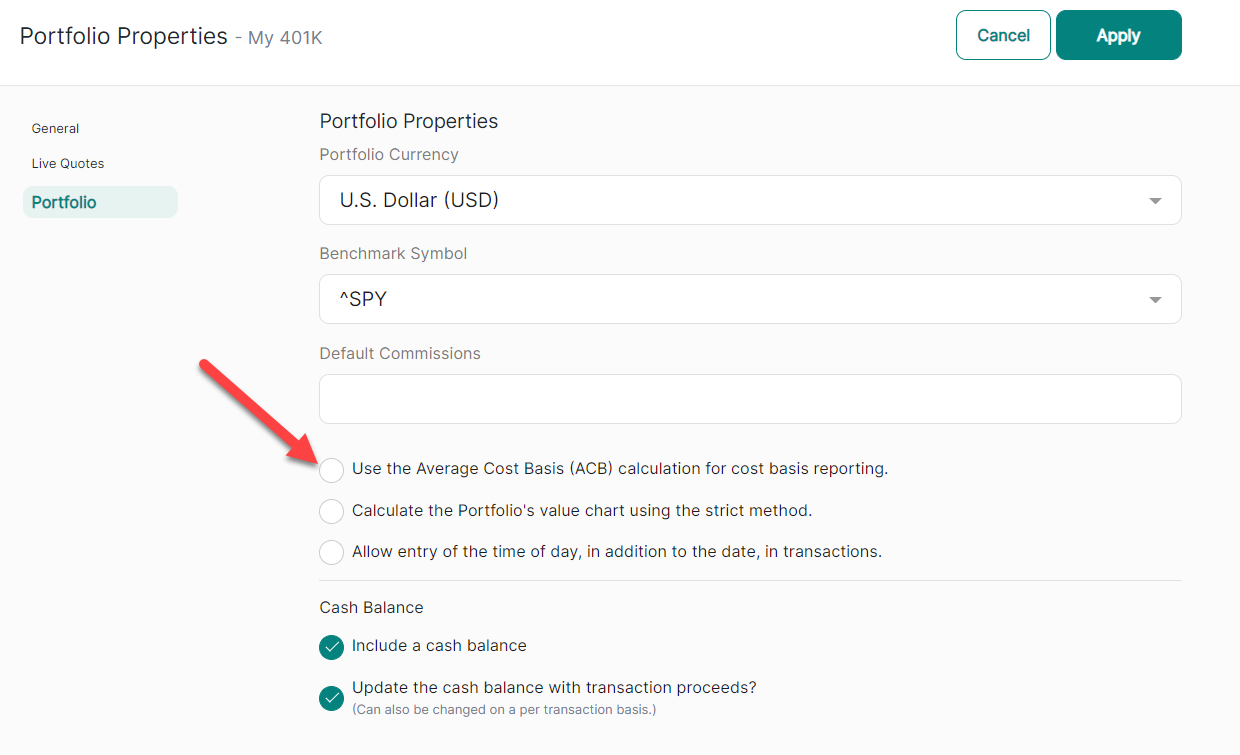# Cost Basis Calculations

The cost basis of your investment is its original value, including commissions and fees, adjusted for splits and distributions. The cost basis value is often referred to as “tax basis” value because it is used to determine your tax obligations.

Re-invested distributions (dividends and capital gains) change your cost basis by adding new lots to your holding. The cost basis value of previously purchased lots of a holding does not change, but the new lots purchased with the distribution proceeds raise the cost basis of your entire investment.

### Calculation Methods

StockMarketEye supports 2 methods of cost basis calculation:

1. Specific Lot Basis - This is the default way cost basis is calculated in StockMarketEye. Each lot of an investment, whether purchased directly or through re-investment, is priced individually.
2. Average Cost Basis (ACB) - This method adds the cost basis values of each lot to arrive at a total value. The ACB per share value is calculated by dividing the total value by the total number of shares. Adding new lots (through normal purchase or re-invested purchase) changes the current ACB per share calculation. At the time of a sale, the current ACB per share value is used to calculate the cost basis of the sold shares.

### Choosing the Calculation Method for a Portfolio

By default, a portfolio uses the Specific Lot basis calculation method. You can change to use the Average Cost Basis (ACB) calculation method by marking the option on the portfolio’s Properties screen.### Available Columns for Cost Basis Values

#### General Columns

These columns show the cost basis values using the currently selected calculation method (either standard or ACB) for the current portfolio.

• Cost Basis - The total cost basis for the position. It will either be the standard cost basis (SCB) or the average cost basis (ACB), depending on the method selected for this portfolio.
• Cost Basis Per Share - The cost basis per share of the position. It will use either the standard cost basis (SCB) or the average cost basis (ACB), depending on the method selected for this portfolio.

#### Standard Specific Lot Method Columns

These columns show the cost basis values using the default, specific lot calculation method.

• SCB
• SCB Per Share

#### Average Cost Basis (ACB) Method Columns

These columns show the cost basis values using the average cost basis (ACB) calculation method.

• ACB
• ACB Per Share